# Level 2 IMO Question Paper for Class 3 - Set 7

Check these Class 3 IMO Level 2 questions from previous years Maths Olympiad exams.

For our paid members, we offer comprehensive benefits, including access to detailed explanations for all questions in our Chapterwise Test Series and Mock Test Series. Our paid members have the opportunity to attempt more than a dozen tests for each chapter covered in the SOF IMO syllabus for Class 3. They can attempt each test multiple times to enhance their learning experience.Our courses can be bought at our BUY COURSES section.

Our Mock Tests for Class 3 IMO follow the exact pattern of SOF IMO in terms of questions, marking and time alloted for completion. This helps the students to experience of the real time exam environment and assess their exam preparedness. Since we follow the same marking system , the scores obtained at the end of each test will help identify students' weaknesses and assist them in improving.

Olympiadtester has displayed a few Chapterwise tests and Mock tests in the FREE SECTION.

1) How many right angles are there in the figure below?OPTIONS

1. 2
2. 6
3. 4
4. 8

2) The figure below is made up of 1-metre squares. Find the perimeter of the figure.OPTIONS

1.  24 m
2. 16 m
3. 9 m
4. 8 m

3) The figure below is made up of 5 identical rectangles. Find the perimeter of the figure.OPTIONS

1. 160 cm
2. 320 cm
3. 600 cm
4. 800 cm

4) How many right angles are there inside the figure below?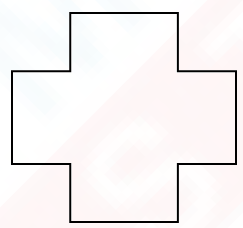OPTIONS

1. 16
2. 12
3. 8
4. 4

5) The area of each square is 4m2. What is the total area of the figure below?OPTIONS

1. 52 m2
2. 48 m2
3. 44 m2
4. 12 m2

6) The figure below is not drawn to scale. Find the perimeter of the figure.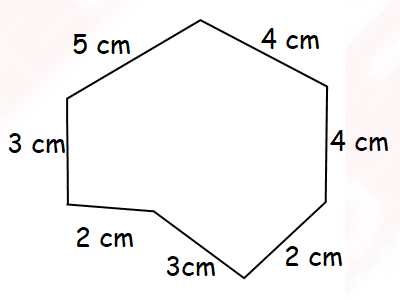OPTIONS

1. 28 cm
2. 25 cm
3. 23 cm
4. 22 cm

7 The figure below shows the layout of Arya's garden. Arya wants to fence the 2 rectangulat flower beds in her garden. ​​​​​​​How much fencing will she require in all?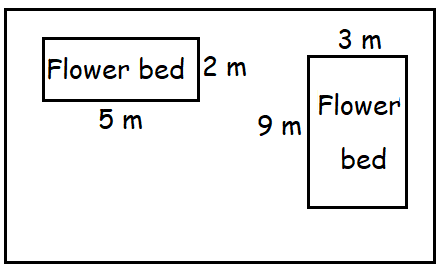OPTIONS

1. 26 m
2. 29 m
3. 38 m
4. 56 m

8) Look at the diagram below, how many pairs of perpendicular lines are there?OPTIONS

1. 1
2. 2
3. 3
4. 4

9) The figure below is not drawn to scale. Find the perimeter of the figure.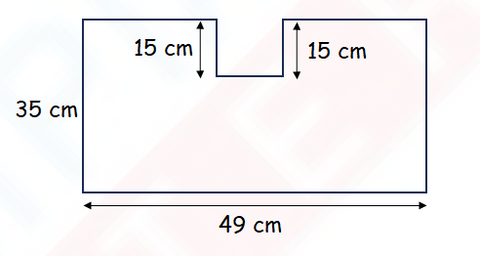OPTIONS

1. 99 cm
2. 129 cm
3. 168 cm
4. 198 cm

10) Look at the figure below. Which pair of lines is parallel?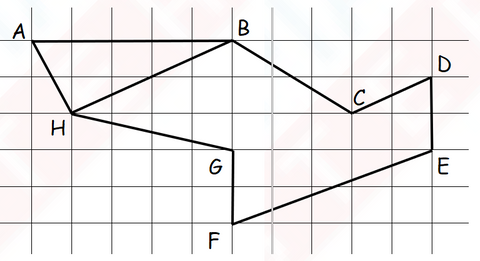OPTIONS

1. HB // CD
2. HG // BC
3. CD // FE
4. AH // BC

10) Figure A is made up of four identical rectangles.The breadth of one rectangle is 3 cm and its length is 3 times of its breadth. Find the perimeter of Figure A.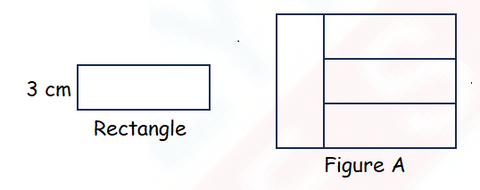11) What is the perimeter of the figure below?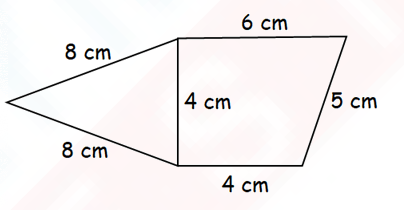OPTIONS

1. 23 cm
2. 29 cm
3. 31 cm
4. 35 cm

12) The total area of a square of side 2 cm and a rectangle is 24 cm2. The length of the side of the square is half the breadth of the rectangle Find the perimeter of the rectangle.

Ans: _______________ cm

13) Find the perimeter of the figure below.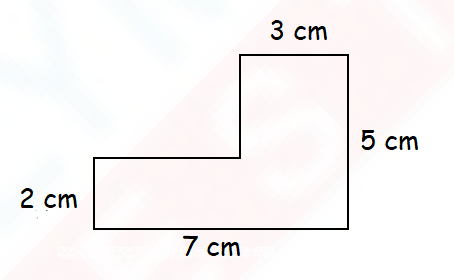Ans: _______________ cm

14) Study the figure below. Which are the 2 angles that are each smaller than a right angle?

Ans: Angle _______ and Angle ________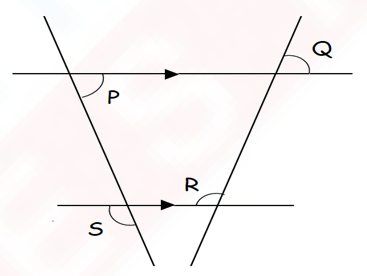15) How many angles inside the figure below are greater than a right angle?
Ans: _______________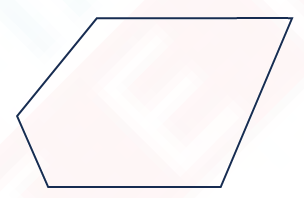Olympiadtester has displayed a few Chapterwise tests and Mock tests in the FREE SECTION.